# RD Sharma Solutions for Class 6 Chapter 7: Decimals Exercise 7.6

Exercise 7.6 has questions which help students understand the uses of decimal notation in money values, measures of lengths, measures of weights, measures of capacity, etc. RD Sharma Solutions Class 6 has answers with explanations in interactive manner to make concepts easier for the students. In order to understand more about the concepts, the students can download RD Sharma Solutions for Class 6 Maths Chapter 7 Decimals Exercise 7.6 PDF which are provided here.

## RD Sharma Solutions for Class 6 Chapter 7: Decimals Exercise 7.6 Download PDF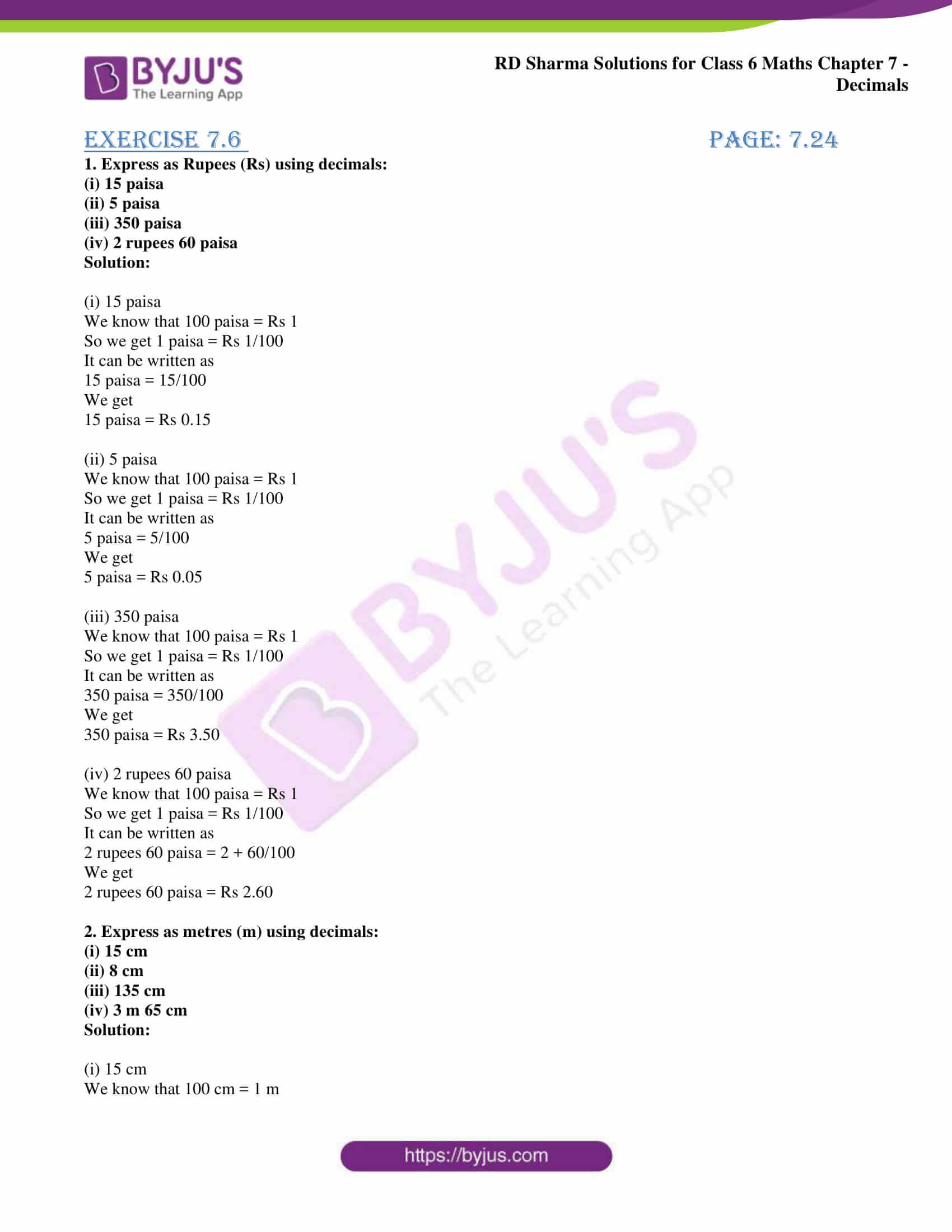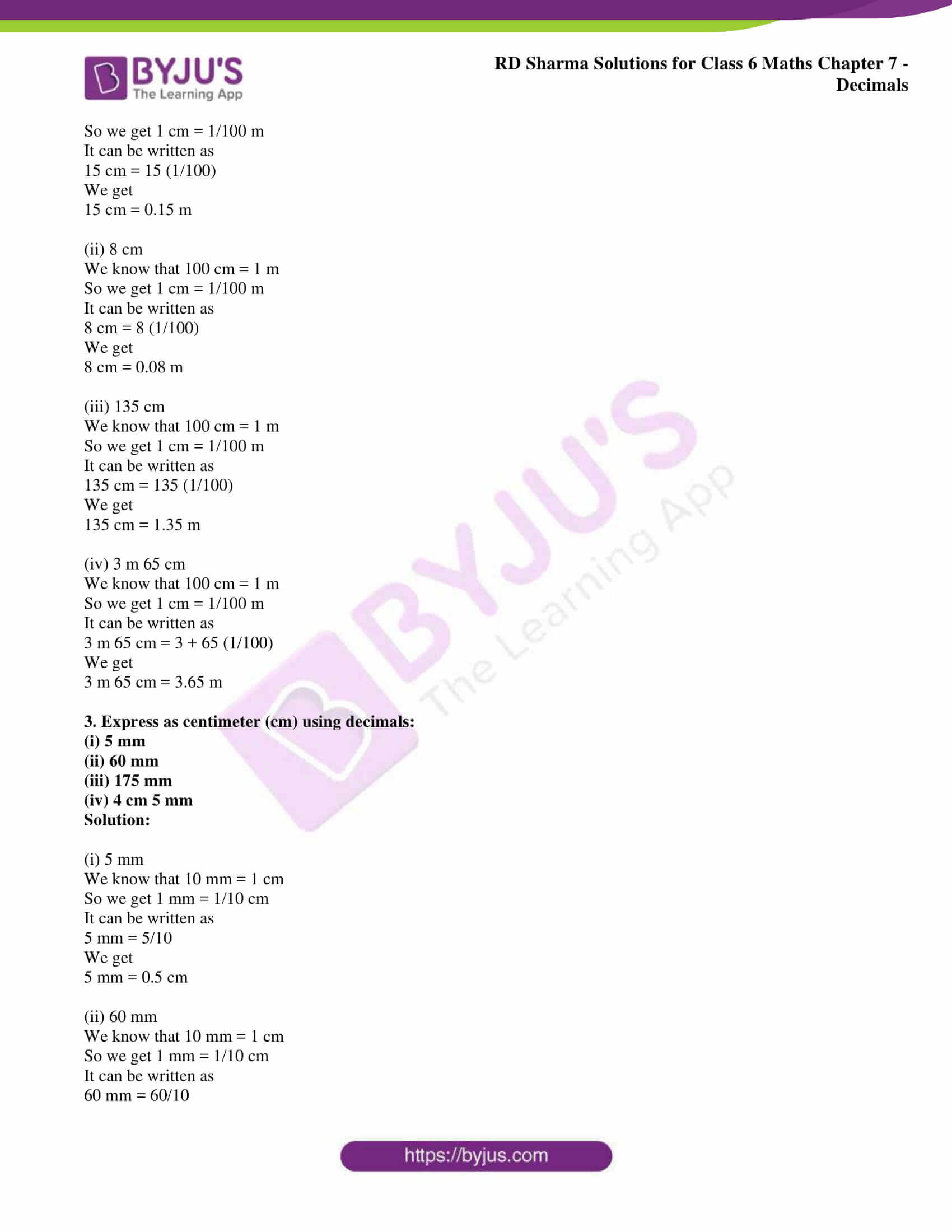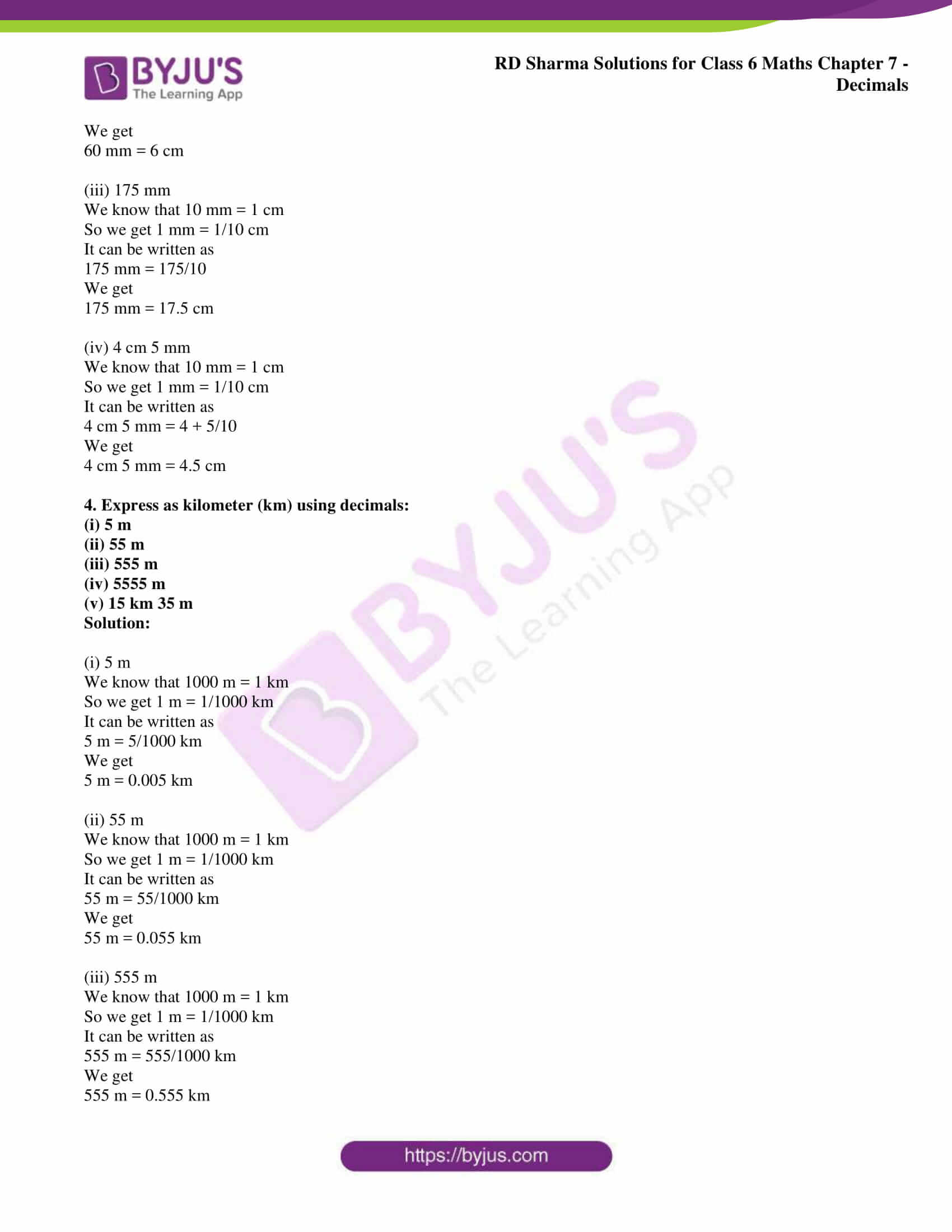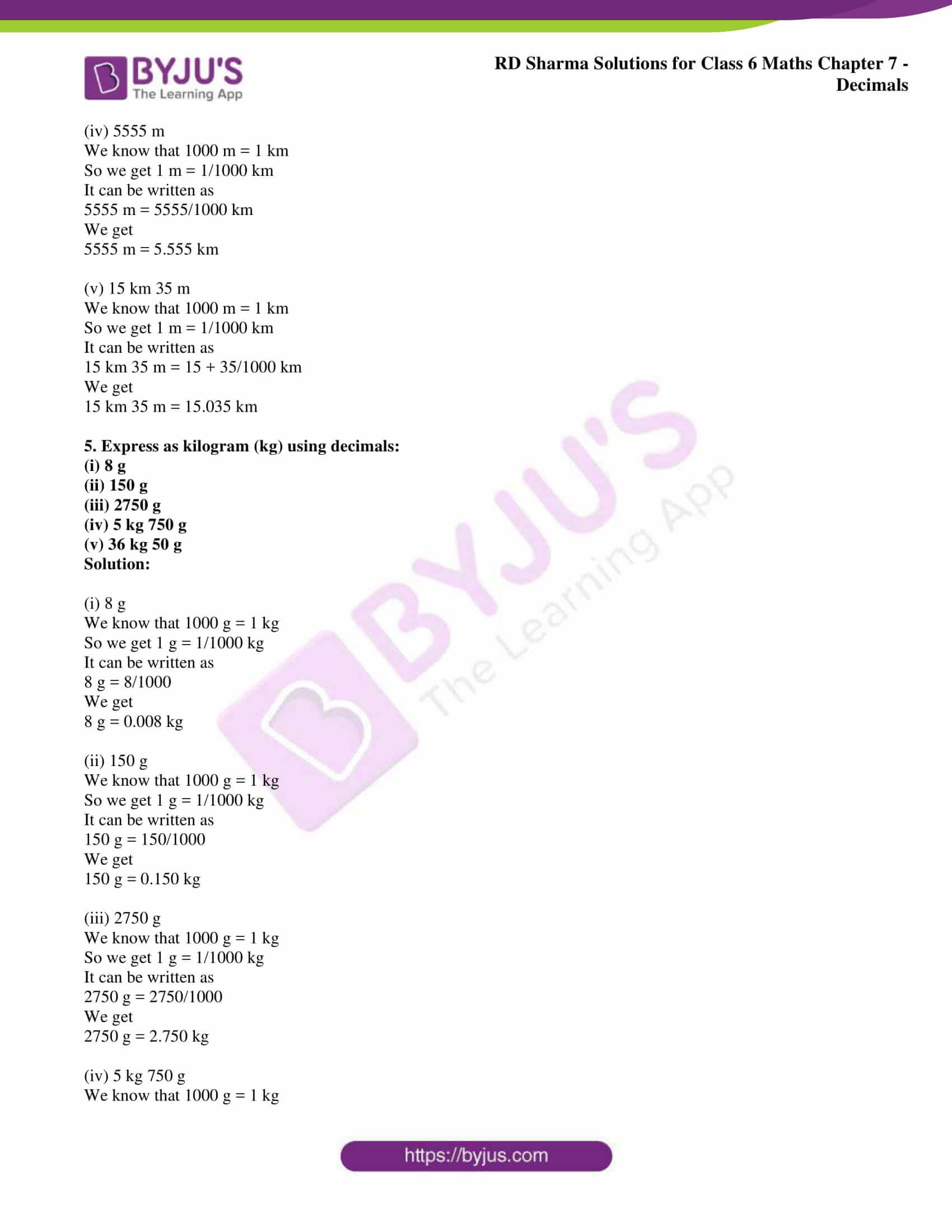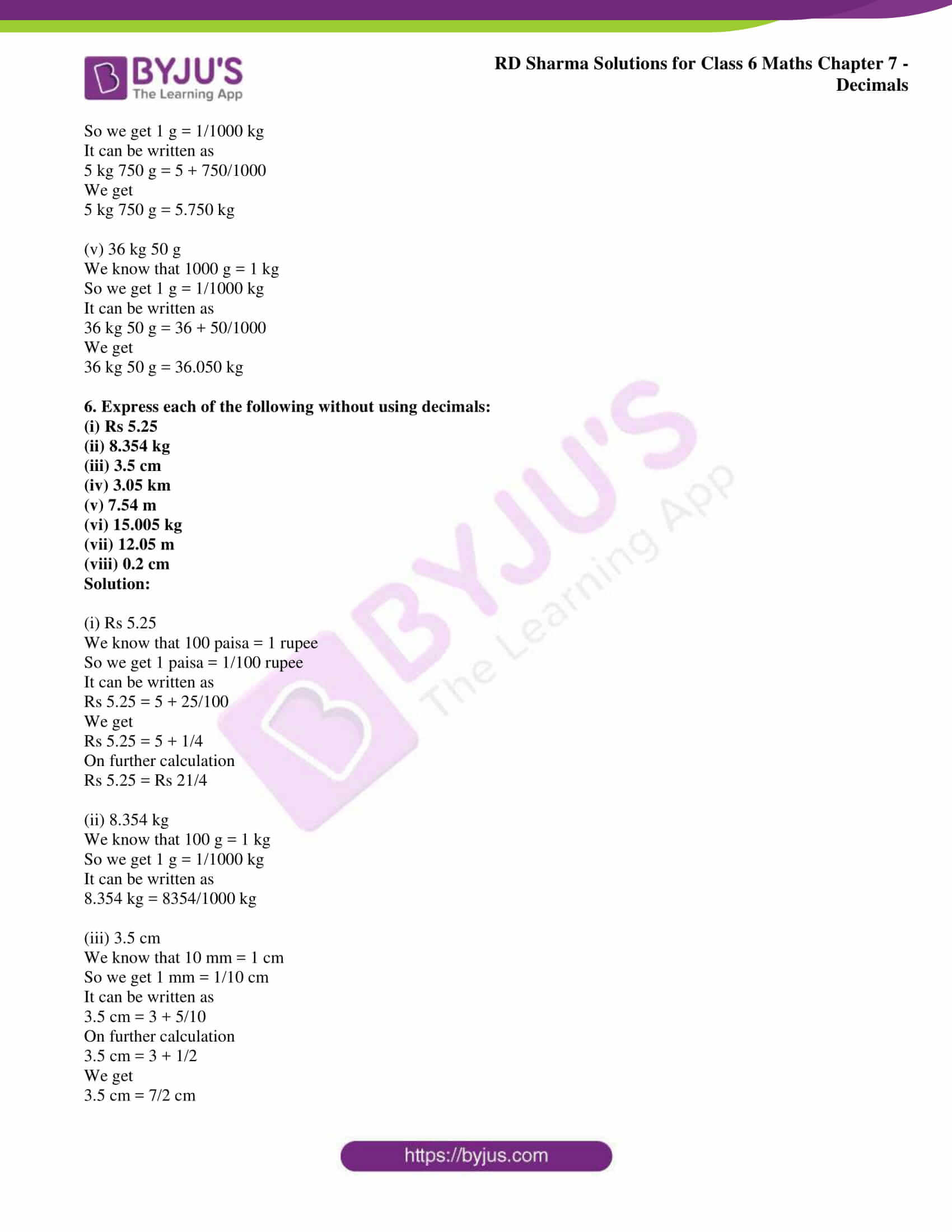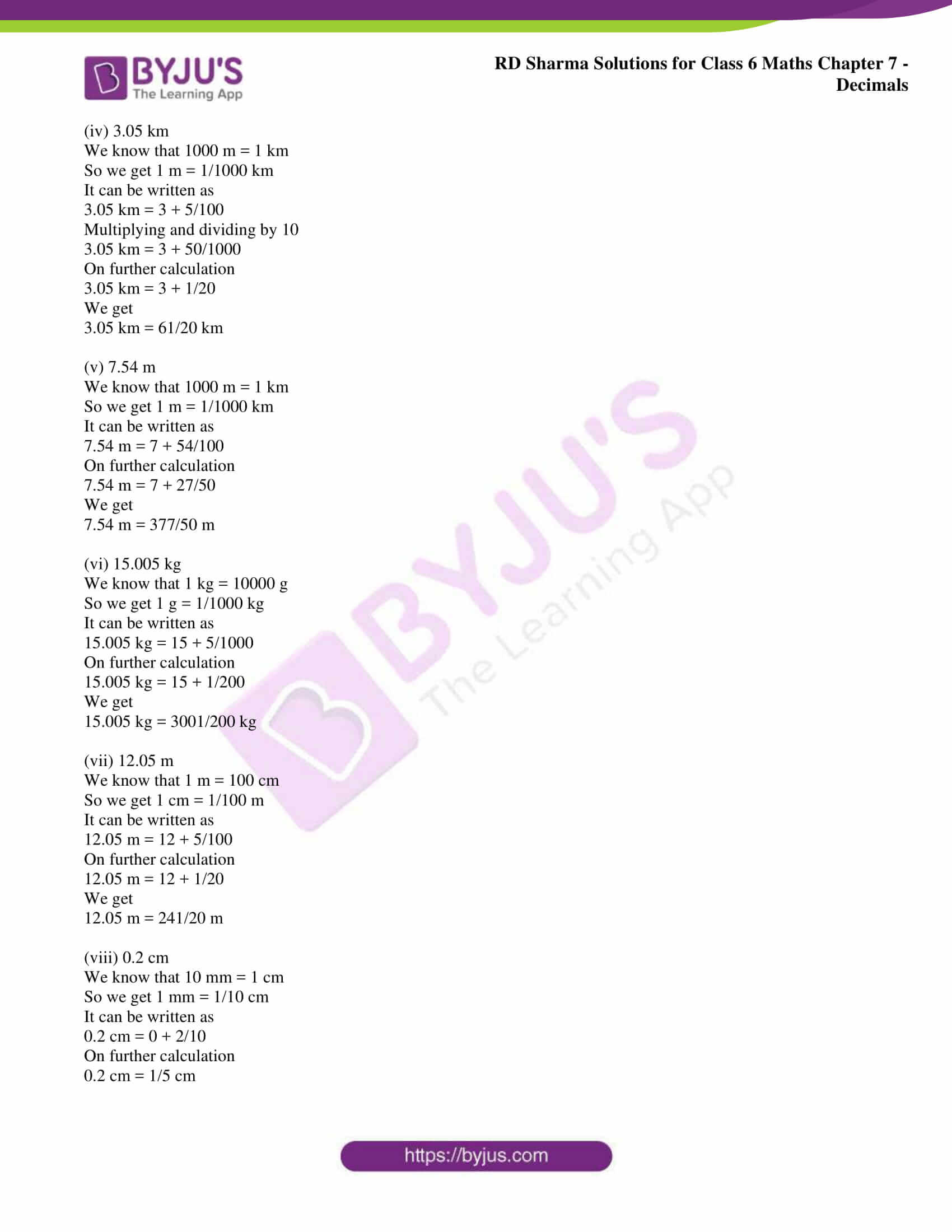### Access RD Sharma Solutions for Class 6 Chapter 7: Decimals Exercise 7.6

1. Express as Rupees (Rs) using decimals:

(i) 15 paisa

(ii) 5 paisa

(iii) 350 paisa

(iv) 2 rupees 60 paisa

Solution:

(i) 15 paisa

We know that 100 paisa = Rs 1

So we get 1 paisa = Rs 1/100

It can be written as

15 paisa = 15/100

We get

15 paisa = Rs 0.15

(ii) 5 paisa

We know that 100 paisa = Rs 1

So we get 1 paisa = Rs 1/100

It can be written as

5 paisa = 5/100

We get

5 paisa = Rs 0.05

(iii) 350 paisa

We know that 100 paisa = Rs 1

So we get 1 paisa = Rs 1/100

It can be written as

350 paisa = 350/100

We get

350 paisa = Rs 3.50

(iv) 2 rupees 60 paisa

We know that 100 paisa = Rs 1

So we get 1 paisa = Rs 1/100

It can be written as

2 rupees 60 paisa = 2 + 60/100

We get

2 rupees 60 paisa = Rs 2.60

2. Express as metres (m) using decimals:

(i) 15 cm

(ii) 8 cm

(iii) 135 cm

(iv) 3 m 65 cm

Solution:

(i) 15 cm

We know that 100 cm = 1 m

So we get 1 cm = 1/100 m

It can be written as

15 cm = 15 (1/100)

We get

15 cm = 0.15 m

(ii) 8 cm

We know that 100 cm = 1 m

So we get 1 cm = 1/100 m

It can be written as

8 cm = 8 (1/100)

We get

8 cm = 0.08 m

(iii) 135 cm

We know that 100 cm = 1 m

So we get 1 cm = 1/100 m

It can be written as

135 cm = 135 (1/100)

We get

135 cm = 1.35 m

(iv) 3 m 65 cm

We know that 100 cm = 1 m

So we get 1 cm = 1/100 m

It can be written as

3 m 65 cm = 3 + 65 (1/100)

We get

3 m 65 cm = 3.65 m

3. Express as centimeter (cm) using decimals:

(i) 5 mm

(ii) 60 mm

(iii) 175 mm

(iv) 4 cm 5 mm

Solution:

(i) 5 mm

We know that 10 mm = 1 cm

So we get 1 mm = 1/10 cm

It can be written as

5 mm = 5/10

We get

5 mm = 0.5 cm

(ii) 60 mm

We know that 10 mm = 1 cm

So we get 1 mm = 1/10 cm

It can be written as

60 mm = 60/10

We get

60 mm = 6 cm

(iii) 175 mm

We know that 10 mm = 1 cm

So we get 1 mm = 1/10 cm

It can be written as

175 mm = 175/10

We get

175 mm = 17.5 cm

(iv) 4 cm 5 mm

We know that 10 mm = 1 cm

So we get 1 mm = 1/10 cm

It can be written as

4 cm 5 mm = 4 + 5/10

We get

4 cm 5 mm = 4.5 cm

4. Express as kilometer (km) using decimals:

(i) 5 m

(ii) 55 m

(iii) 555 m

(iv) 5555 m

(v) 15 km 35 m

Solution:

(i) 5 m

We know that 1000 m = 1 km

So we get 1 m = 1/1000 km

It can be written as

5 m = 5/1000 km

We get

5 m = 0.005 km

(ii) 55 m

We know that 1000 m = 1 km

So we get 1 m = 1/1000 km

It can be written as

55 m = 55/1000 km

We get

55 m = 0.055 km

(iii) 555 m

We know that 1000 m = 1 km

So we get 1 m = 1/1000 km

It can be written as

555 m = 555/1000 km

We get

555 m = 0.555 km

(iv) 5555 m

We know that 1000 m = 1 km

So we get 1 m = 1/1000 km

It can be written as

5555 m = 5555/1000 km

We get

5555 m = 5.555 km

(v) 15 km 35 m

We know that 1000 m = 1 km

So we get 1 m = 1/1000 km

It can be written as

15 km 35 m = 15 + 35/1000 km

We get

15 km 35 m = 15.035 km

5. Express as kilogram (kg) using decimals:

(i) 8 g

(ii) 150 g

(iii) 2750 g

(iv) 5 kg 750 g

(v) 36 kg 50 g

Solution:

(i) 8 g

We know that 1000 g = 1 kg

So we get 1 g = 1/1000 kg

It can be written as

8 g = 8/1000

We get

8 g = 0.008 kg

(ii) 150 g

We know that 1000 g = 1 kg

So we get 1 g = 1/1000 kg

It can be written as

150 g = 150/1000

We get

150 g = 0.150 kg

(iii) 2750 g

We know that 1000 g = 1 kg

So we get 1 g = 1/1000 kg

It can be written as

2750 g = 2750/1000

We get

2750 g = 2.750 kg

(iv) 5 kg 750 g

We know that 1000 g = 1 kg

So we get 1 g = 1/1000 kg

It can be written as

5 kg 750 g = 5 + 750/1000

We get

5 kg 750 g = 5.750 kg

(v) 36 kg 50 g

We know that 1000 g = 1 kg

So we get 1 g = 1/1000 kg

It can be written as

36 kg 50 g = 36 + 50/1000

We get

36 kg 50 g = 36.050 kg

6. Express each of the following without using decimals:

(i) Rs 5.25

(ii) 8.354 kg

(iii) 3.5 cm

(iv) 3.05 km

(v) 7.54 m

(vi) 15.005 kg

(vii) 12.05 m

(viii) 0.2 cm

Solution:

(i) Rs 5.25

We know that 100 paisa = 1 rupee

So we get 1 paisa = 1/100 rupee

It can be written as

Rs 5.25 = 5 + 25/100

We get

Rs 5.25 = 5 + 1/4

On further calculation

Rs 5.25 = Rs 21/4

(ii) 8.354 kg

We know that 100 g = 1 kg

So we get 1 g = 1/1000 kg

It can be written as

8.354 kg = 8354/1000 kg

(iii) 3.5 cm

We know that 10 mm = 1 cm

So we get 1 mm = 1/10 cm

It can be written as

3.5 cm = 3 + 5/10

On further calculation

3.5 cm = 3 + 1/2

We get

3.5 cm = 7/2 cm

(iv) 3.05 km

We know that 1000 m = 1 km

So we get 1 m = 1/1000 km

It can be written as

3.05 km = 3 + 5/100

Multiplying and dividing by 10

3.05 km = 3 + 50/1000

On further calculation

3.05 km = 3 + 1/20

We get

3.05 km = 61/20 km

(v) 7.54 m

We know that 1000 m = 1 km

So we get 1 m = 1/1000 km

It can be written as

7.54 m = 7 + 54/100

On further calculation

7.54 m = 7 + 27/50

We get

7.54 m = 377/50 m

(vi) 15.005 kg

We know that 1 kg = 10000 g

So we get 1 g = 1/1000 kg

It can be written as

15.005 kg = 15 + 5/1000

On further calculation

15.005 kg = 15 + 1/200

We get

15.005 kg = 3001/200 kg

(vii) 12.05 m

We know that 1 m = 100 cm

So we get 1 cm = 1/100 m

It can be written as

12.05 m = 12 + 5/100

On further calculation

12.05 m = 12 + 1/20

We get

12.05 m = 241/20 m

(viii) 0.2 cm

We know that 10 mm = 1 cm

So we get 1 mm = 1/10 cm

It can be written as

0.2 cm = 0 + 2/10

On further calculation

0.2 cm = 1/5 cm